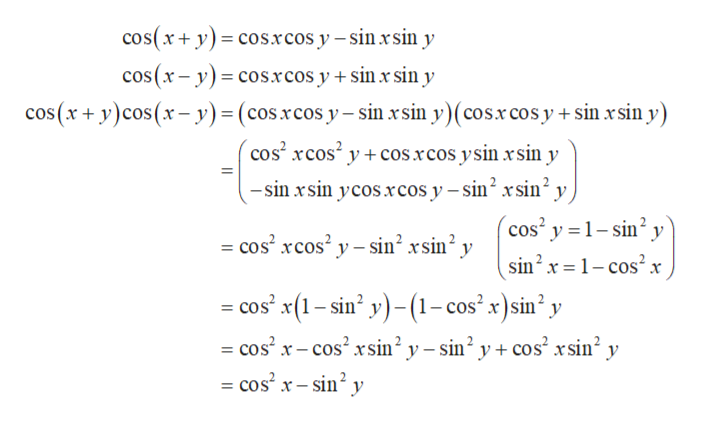Question
18 views

Verify the tirgonometric identity.

cos(x+y)cos(x-y)=cos^2x-sin^2y

check_circle

Step 1

The given expression to be proved is,

Step 2

LHS can be evaluated as,help_outlineImage Transcriptionclosecos(xy) cosxcos y -sin xsin y cos(x-y) cosrcos y + sin x sin y cos(x+ y)cos(x-y)= (cosxcos y-sin xsin y)( cosx cos y + sin x sin y) cos rcos2 ycos .xcos ysin xsin y -sin rsin ycos xcos y - sin2 xsin2 y, 'cos2 y 1-sin2 y cos xcos2 y-sin xsin2 y sin2 x1-cos2 x = cos' x(1-sin' y)-(1- cos' x)sin' y cos x-cos sin2y- sin2 y+cos' rsin2 y cos2 x-sin2 y fullscreen
Step 3

RHS can be writ...

### Want to see the full answer?

See Solution

#### Want to see this answer and more?

Solutions are written by subject experts who are available 24/7. Questions are typically answered within 1 hour.*

See Solution
*Response times may vary by subject and question.
Tagged in

### Trigonometry